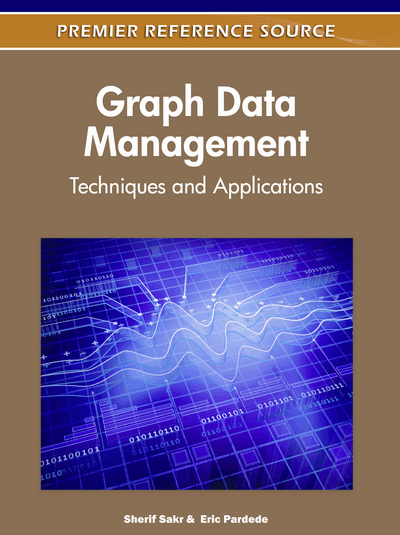# Clustering Vertices in Weighted Graphs

Derry Tanti Wijaya (Carnegie Mellon University, USA) and Stephane Bressan (National University of Singapore, Singapore)
Copyright: © 2012 |Pages: 14
DOI: 10.4018/978-1-61350-053-8.ch012
OnDemand PDF Download:
Available
\$37.50
No Current Special Offers

## Abstract

Clustering is the unsupervised process of discovering natural clusters so that objects within the same cluster are similar and objects from different clusters are dissimilar. In clustering, if similarity relations between objects are represented as a simple, weighted graph where objects are vertices and similarities between objects are weights of edges; clustering reduces to the problem of graph clustering. A natural notion of graph clustering is the separation of sparsely connected dense sub graphs from each other based on the notion of intra-cluster density vs. inter-cluster sparseness. In this chapter, we overview existing graph algorithms for clustering vertices in weighted graphs: Minimum Spanning Tree (MST) clustering, Markov clustering, and Star clustering. This includes the variants of Star clustering, MST clustering and Ricochet.
Chapter Preview
Top

## Introduction

Graphs are mathematical structures used to represent pairwise relations between objects from a certain collection. Graphs contain vertices (that represent objects) and edges connecting the vertices (that represent pairwise relations between the objects). A graph G is therefore an ordered pair G = (V, E) where V is a set of vertices and E is a multiset of edges: unordered pairs of vertices. A simple, weighted graph is an undirected graph that has no self-loops and no more than one edge between any two vertices: i.e. edges form a set rather than a multiset and each edge is an unordered pair of distinct vertices. A number or weight is assigned to each edge, representing the weight of relation between the two vertices connected by the edge.

Clustering is the unsupervised process of discovering natural clusters so that objects within the same cluster are similar and objects from different clusters are dissimilar according to some similarity measurements. In a vector space, for instance, where objects are represented by feature vectors, similarity is generally defined as the cosine of the angle between vectors. In clustering, if similarity relations between objects are represented as a simple, weighted graph where objects are vertices and similarities between objects are weights of edges; clustering reduces to the problem of clustering vertices in a weighted graph. A natural notion of graph clustering is the separation of sparsely connected dense sub graphs from each other based on the notion of intra-cluster density vs. inter-cluster sparseness. Clustering is the separation of dense from sparse regions of space or components of graphs. Clusters are the dense regions or components.

In this chapter, we first identify graph algorithms related to clustering problem: random walk and minimum spanning tree algorithms. Intuitively, random walk algorithm can produce ranking of vertices in the graph thus identify potentially significant vertices. Minimum spanning tree algorithm can identify potentially significant edges or path in the graph. These issues are closely related to the problem of clustering: identification of significant vertices as candidate centroids (seeds) and the identification of significant edges to merge or split clusters. We present an overview of existing graph algorithms for clustering vertices in weighted graphs: Minimum Spanning Tree (MST) clustering, Markov clustering, and Star clustering. We also present novel family of Star clustering variants, MST clustering variants we call MST-Sim, and Ricochet.

Our variants of Star clustering explore various techniques, including random walk algorithm, to identify centroids (Star centers). MST-Sim explores minimum spanning tree algorithm for clustering, adding to it intra and inter-cluster similarity metrics that have basis in graph theory. Ricochet uses results of our study on Star clustering to identify centroids and our study of minimum spanning tree algorithm to identify edges to merge clusters. Ricochet also uses the idea of vertices reassignment from K-means to reach terminating condition. Combining these ideas results in Ricochet being unconstrained – it does not require any parameter to be defined a priori – and able to maximize intra-cluster similarities

## Complete Chapter List

Search this Book:
Reset